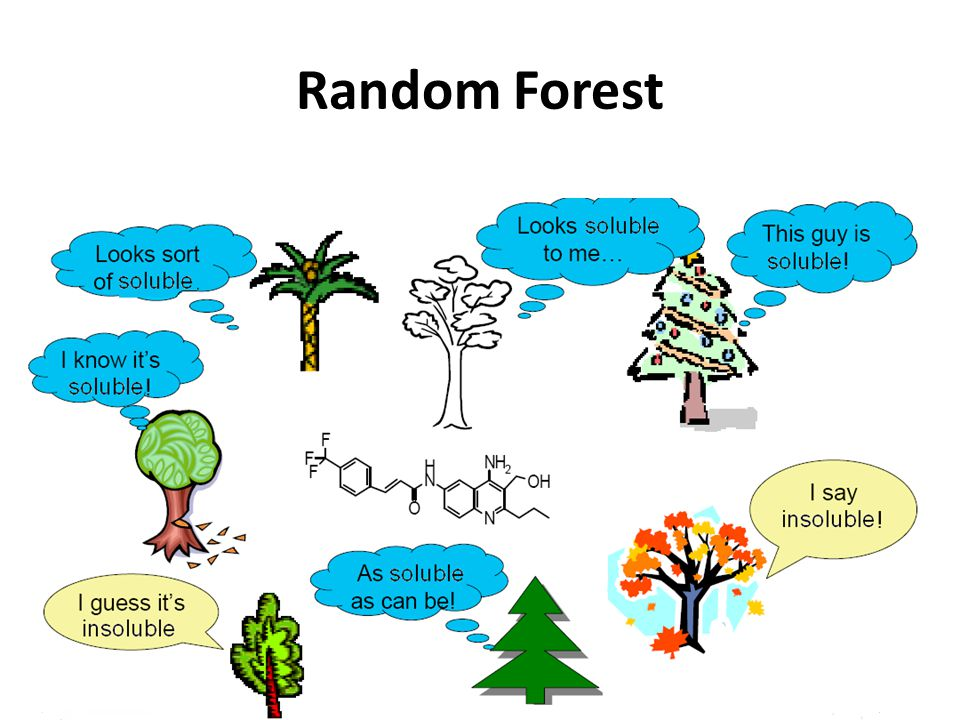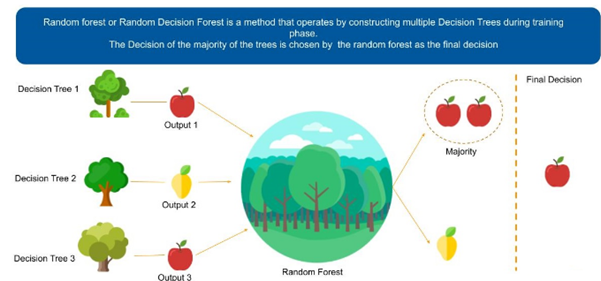# Random Forest-Theory

### Random Forest-Theoryimage source – google.com

Random forest algorithm is a supervised algorithm. As you can guess from its name this algorithm creates a forest with number of trees. It operates by constructing multiple decision trees. The final decision is made based on the majority of the trees and is chosen by the random forest.image source – google.com

The method of combining trees is known as an ensemble method. Ensembling is nothing but a combination of weak learners (individual trees) to produce a strong learner.

Let’s understand ensemble with an example. Let’s suppose you want to watch movie but you have doubt in your mind regarding it’s reviews, so you have asked 10 people who have watched the movie, 8 of them said movie is fantastic and 2 of them said movie was not good. Since the majority is in favour, you decide to watch the movie. This is how we use ensemble techniques in our daily life too.

Random Forest can be used to solve regression and classification problems. In regression problems, the dependent variable is continuous. In classification problems, the dependent variable is categorical.

### Advantages and Disadvantages of Random Forest

#### Advantages are as follows:

1. It is used to solve both regression and classification problems.
2. It can be also used to solve unsupervised ML problems.
3. It can handle thousands of input variables without variable selection.
4. It can be used as a feature selection tool using its variable importance plot.
5. It takes care of missing data internally in an effective manner.

#### Disadvantages are as follows:

1. This is a black-box model so Random Forest model is difficult to interpret.
2. It can take longer than expected time to computer a large number of trees.

### How Random Forest works?

#### Algorithm can be divided into two stages.

• Random forest creation.
• Perform prediction from the created random forest classifier.

#### Random forest creation:

To create random forest we need to select following steps

1. Randomly select “k” features from total “m” features, where k << m.
2. Among the “k” features, calculate the node “d” using the best split point.
3. Split the node into child nodes using the best split.
4. Repeat 1 to 3 steps until “L” number of nodes has been reached.
5. Build forest by repeating steps 1 to 4 for “n” number times to create “n” number of trees.

#### Perform prediction from the created random forest classifier

To perform prediction we need to take following steps

1. Takes the test features and use the rules of each randomly created decision tree to predict the outcomes and stores the predicted outcome (target)
2. Calculate the votes for each predicted target.
3. Consider the high voted predicted target as the final prediction from the random forest algorithm.

### Set the parameters for the random forest model:

Parameters = {‘bootstrap’: True,’min_samples_leaf’: 3, ‘n_estimators’: 50, ‘min_samples_split’: 10, ‘max_features’: ‘sqrt’,’max_depth’: 6,’max_leaf_nodes’: None}

### Hyperparameters Tuning of Random forest classifier:

#### min_samples_leaf : int, float, optional (default=1)

The minimum number of samples required to be at a leaf node:

• If int, then consider min_samples_leaf as the minimum number.
• If float, then min_samples_leaf is a percentage and ceil(min_samples_leaf * n_samples) are the minimum number of samples for each node.

#### n_estimators : integer, optional (default=10):

• The number of trees in the forest.

#### min_samples_split: int, float, optional (default=2):

The minimum number of samples required to split an internal node:

• If int, then consider min_samples_split as the minimum number.
• If float, then min_samples_split is a percentage and ceil(min_samples_split * n_samples) are the minimum number of samples for each split.

#### max_features : int, float, string or None, optional (default=”auto”):

The number of features to consider when looking for the best split:

• If int, then consider max_features features at each split. -If float, then max_features is a percentage and int(max_features * n_features) features are considered at each split.
• If “auto”, then max_features=sqrt(n_features).
• If “sqrt”, then max_features=sqrt(n_features) (same as “auto”).
• If “log2”, then max_features=log2(n_features).
• If None, then max_features=n_features.

#### max_depth : integer or None, optional (default=None):

The maximum depth of the tree. If None, then nodes are expanded until all leaves are pure or until all leaves contain less than min_samples_split samples.

#### max_leaf_nodes : int or None, optional (default=None):

Grow trees with max_leaf_nodes in best-first fashion. Best nodes are defined as relative reduction in impurity. If None then unlimited number of leaf nodes.

If you want to learn more about the rest of hyperparameters , check here